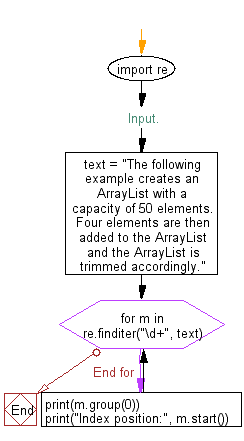﻿ Python Exercises: Separate and print the numbers and their position of a given string - w3resource# Python: Separate and print the numbers and their position of a given string

## Python Regular Expression: Exercise-29 with Solution

Write a Python program to separate and print the numbers and their position of a given string.

Sample Solution:-

Python Code:

``````import re
# Input.
text = "The following example creates an ArrayList with a capacity of 50 elements. Four elements are then added to the ArrayList and the ArrayList is trimmed accordingly."

for m in re.finditer("\d+", text):
print(m.group(0))
print("Index position:", m.start())
```
```

Sample Output:

```50
Index position: 62
```

Pictorial Presentation:Flowchart:## Visualize Python code execution:

The following tool visualize what the computer is doing step-by-step as it executes the said program:

Python Code Editor:

Have another way to solve this solution? Contribute your code (and comments) through Disqus.

What is the difficulty level of this exercise?

Test your Python skills with w3resource's quiz

﻿

## Python: Tips of the Day

Creates a dictionary with the same keys as the provided dictionary and values generated by running the provided function for each value

Example:

```def tips_map_values(obj, fn):
ret = {}
for key in obj.keys():
ret[key] = fn(obj[key])
return ret
users = {
'Owen': { 'user': 'Owen', 'age': 29 },
'Eddie': { 'user': 'Eddie', 'age': 15 }
}

print(tips_map_values(users, lambda u : u['age'])) # {'Owen': 29, 'Eddie': 15}
```

Output:

```{'Owen': 29, 'Eddie': 15}
```